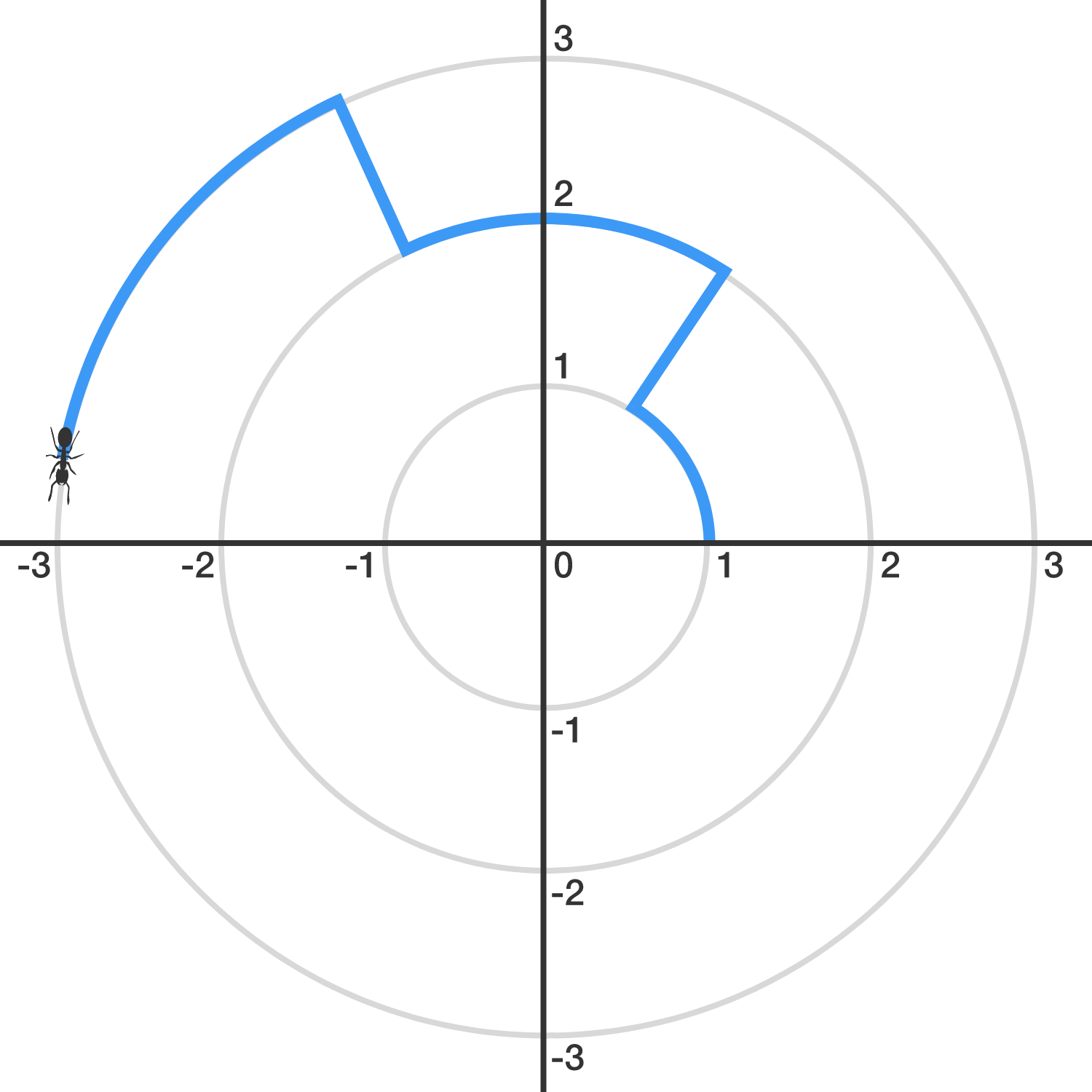Geometry

# Trigonometric Functions: Level 3 Challenges

Find the value of $-\sin^2{1^\circ}+\sin^2{2^\circ }-\sin^2{3^\circ }+\ldots+\sin^2{88^\circ}-\sin^2{89^\circ}+\sin^2{90^\circ}$

$\left [ \log_{10} \left ( \tan \left ( 1^\circ \right ) \right ) \right ] \times \left [ \log_{10} \left ( \tan \left ( 2^\circ \right ) \right ) \right ] \times \left [ \log_{10} \left ( \tan \left ( 3^\circ \right ) \right ) \right ] \times \cdot \cdot \cdot \\ \times \left [ \log_{10} \left ( \tan \left ( 88^\circ \right ) \right ) \right ] \times \left [ \log_{10} \left ( \tan \left ( 89^\circ \right ) \right ) \right ] = \ ?$An ant finds itself trapped in the $xy$-plane, and its initial position is $(1,0).$

Let $S_k$ denote the circle with radius $k$ centered around the origin. Starting from $(1,0)$, the ant walks 1 unit counter-clockwise on $S_{1}.$ Then, it walks directly (radially outward) to $S_2,$ on which it will walk 2 units counter-clockwise. Then, it will walk directly to $S_3$ and walk 3 units counter-clockwise, and so, with the ant walking $k$ units on $S_k.$ (See the image above.)

When the ant crosses the positive $x$-axis for the first time since it left $(1,0)$, it is on $S_{n}$. What is $n$?

Find the value of

$\frac{\cos^4 75^{\circ}+\sin^4 75^{\circ}+3\sin^2 75^{\circ}\cos^2 75^{\circ}}{\cos^6 75^{\circ}+\sin^6 75^{\circ}+4\sin^2 75^{\circ}\cos^2 75^{\circ}}.$

If the domain of $f(x)=\tan^{-1} \left( \dfrac{x^2+1}{x^2+\sqrt{3}}\right)$ is the real numbers, find its range.

×

Problem Loading...

Note Loading...

Set Loading...